# From Analogue To Digital Effects

Synth Secrets By Gordon Reid
Published April 2004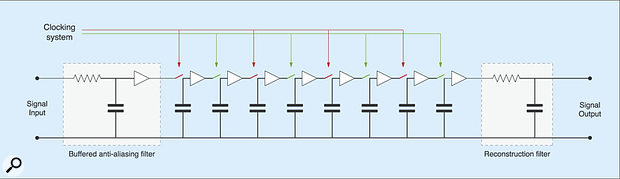Figure 1: A simple eight-stage bucket-brigade device (or BBD) delay line.

When synthesizing sounds, the effects you place after your synth's output are often as important as the synth itself (just think of last month's Leslie). As we near the end of Synth Secrets, we consider how a digital effects processor works.

Last month, as part of the final push to synthesize the effects of the Leslie rotary speaker, I introduced the bucket-brigade device (or BBD) delay line and showed how we could attempt to use this to assist the simulation. I then showed that, while possible, this was not practical. In fact, because of space considerations, I omitted a number of secondary factors that make analogue recreations of the Leslie less than satisfactory. For example, the spatial amplitude response of the horn assembly is not smooth, so the volume of the sound 'wobbles' as lobes of loudness and quietness rotate past your ears. What's more, this response is frequency-dependent, meaning that there are independent amplitude modulations occurring for each frequency in the signal. Then there's the bass rotor... Due to its limited size, this is not effective at frequency-modulating low-frequency signal components so, unlike the horn, it is more a source of amplitude modulation ('tremolo') than frequency modulation.

All in all, it's little wonder that I gave up on my quest for the 'Analogue Leslie' and sent you away to find a Korg G4 or some other low-cost digital Leslie simulator. Admittedly, there are many simpler effects for which the perceived 'warmth' of analogue electronics is a bonus.

For example, I don't think that anybody has improved upon the Electro-harmonix Deluxe Electric Mistress or MXR Flanger/Doubler, and even low-cost stomp boxes such as the Small Stone phaser and Big Muff occupy a unique place... often emulated, but only equalled if copied almost component for component. Nevertheless, for emulations of rotary speakers and the creation of new and esoteric effects, digital electronics is king. So, given that we're now nearing the end of our journey through the world of synthesis — from oscillators and filters at one end to the effects at the other — I think that it's time to introduce the fundamental electronic concepts that make digital audio possible.

## Analogue To Digital

We'll start by returning to the BBD that I explained last month, and which I've recreated in Figure 1 (above). This shows a signal entering the delay line through an anti-aliasing filter on the left of the diagram, passing through each stage in turn, and then exiting through the reconstruction filter on the right. The length of delay is determined by just two factors; the number of BBD stages and the speed of the clock driving the switches shown in red and green.

As we'll see, the elements that make up the digital equivalent of the analogue delay line are very similar in function. The principles at work in the BBD — of storing a slice of the incoming signal, holding it, and passing it down a line of identical, signal-storing components at a rate determined by a clock — are the same, although of course the means of storing the incoming signal is different. Instead of the signal being stored as a voltage in a capacitor at each stage of the BBD, it's a digital representation of the signal that is stored and processed, a binary number consisting of a string of ones and zeroes.

In order for this to be possible, the input signal has to pass through an analogue-to-digital converter (ADC) as it goes into the effect units. Most of us are now familiar with the concept of how one of these works; the instantaneous signal voltage is measured at intervals determined by the sampling rate (every 1/44100th of a second for CD-quality audio), then that voltage measurement is converted into a binary number composed of bits (the number of digits in the binary number). The greater the number of bits, the higher the resolution of the signal measurement, and all other things being equal, this will enable you to represent the analogue voltage with smaller errors. CD-quality audio is 16-bit, so the voltage measurements are stored as strings of 16 digits, all of which are either a zero or a one.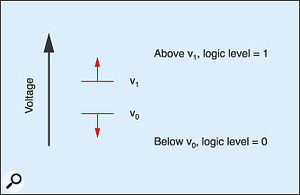Figure 2: The nature of a digital system.I'm now going to explain what happens to a single one of those bits in a digital delay line — just one of the digits that makes up the binary measurement of the original analogue signal in one sample. In short, it passes through the digital equivalent of the BBD, which is known as a 'shift register', and which is itself constructed from devices called logic gates. However, to understand how a shift register works, we must first take a diversion into the fundamental nature of binary numbers and logic. Hold onto your hat... this is going to take us into a fascinating realm of technology not yet plumbed by Synth Secrets. If you'd rather not have your hat disturbed by the finer points of how logic gates and shift registers work, you can skip ahead to the 'An Audio Delay Line' section.

## Combinational Logic

Firstly, it's all very well to say that the analogue signal is converted by the ADC into a string of ones and zeroes, but what does that actually mean? After all, how does a '1' or a '0' pass through an electronic circuit? Well, perhaps strangely, given that the input signal started off as a voltage, the zeros and ones that make up a 16-bit sample are also voltages, albeit used differently. In this case, any voltage above a predetermined level 'v1' is said to be a '1', while a voltage below another predetermined level 'v0' is said to be a '0' (see Figure 2).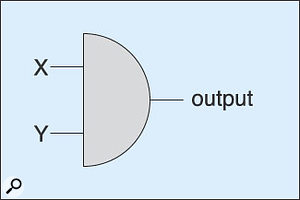Figure 3: An AND gate. Note that the gate symbols I have used in this article are a mixture of traditional British and American standards. You may encounter others elsewhere.

Actually, this is an idealised view, and to make such a system work, the change from '0' to '1' generally occurs when the voltage passes from below v1 to above v1, and a transition from '1' to '0' occurs when the voltage drops from above v0 to below v0. So the 16-bit numbers that are output at each clock step of the ADC are represented by 16 voltages passing through the effects unit, each of which is understood by the circuits through which it passes as a '1' or a '0'. And what are these circuits? As I said earlier, they're logic gates, whose operation is predicated on their ability to determine whether their inputs are in one state or another: zero or one.

One of the simplest forms of logic gate is a device called the two-input AND gate, which I've represented in Figure 3, and whose operation I have described in the 'truth table' shown below. If you look at this, you'll see that both the level presented to input 'X' and the level presented to input 'Y' must be '1' for the output of the device to be a '1'. Any other combination results in an output of '0'. The circuitry required to do this is remarkably simple, but explaining it would take us off into transistor electronics, which is not where I want to go, so we'll say no more about it here.

There are numerous other types of logic gate. For example, there's the OR gate, which, in its two-input form, produces a '1' when either of X or Y are '1' (see Figure 4).

Table 1: The truth table for a two-input AND gate.

 INPUT X INPUT Y OUTPUT 0 0 0 1 0 0 0 1 0 1 1 1

Table 2: The truth table for a two-input OR gate.

 INPUT X INPUT Y OUTPUT 0 0 0 1 0 1 0 1 1 1 1 1

Table 3: The truth table for a NOT gate.

 INPUT X OUTPUT 0 1 1 0

Table 4: The truth table for a two-input NAND gate.

 INPUT X INPUT Y OUTPUT 0 0 1 1 0 1 0 1 1 1 1 0

Table 5: The truth table for a two-input NOR gate.

 INPUT X INPUT Y OUTPUT 0 0 1 1 0 0 0 1 0 1 1 0

Table 6: The truth table for a two-input XOR gate.

 INPUT X INPUT Y OUTPUT 0 0 0 1 0 1 0 1 1 1 1 0

Table 7: The truth table for a two-input XNOR gate.

 INPUT X INPUT Y OUTPUT 0 0 1 1 0 0 0 1 0 1 1 1

Next comes the NOT gate, or 'inverter' which has a single input, and produces a '0' when the input is '1', and vice versa (see Figure 5).

If you consider the action of an AND followed by a NOT, it follows that there is another device, called a NAND gate, that responds to the logic 'and not', meaning that a '1' is output when [input X and input Y] is not '1' (see Figure 6).

Likewise, there is a NOR gate which acts as an OR followed by a NOT (see Figure 7).

Next, there's the XOR ('exclusive OR') gate, which produces a '1' when either X or Y are '1', but not when both are '1' (see Figure 8, below).

Finally, there's the XNOR, which is the inverse of the XOR. This outputs '1's when the inputs are both '0' or both '1', but not otherwise (see Figure 9, below).

Once you have these seven devices at your disposal, you can design anything from a simple logic switch to the most complex computer. You don't even need all seven types because, with just AND, NOT and OR, you can derive all the others. Although this may not be the most efficient way to obtain a given result, it means that many complex problems can be reduced to a simpler form.

## Gates With Memory

What we have discussed so far is called 'combinational logic' because the inputs at any moment determine the values of the outputs. In other words, individual gates and many of the systems developed from them have no 'memory'.

Fortunately, we can build a 'sequential circuit' — one whose output is not only dependent upon the current inputs but also on past inputs — by connecting the outputs of two NAND gates to each other's inputs. This forms a feedback loop that, for a given set of inputs, snaps into one state or another, and then holds this state after the inputs are removed. The circuit thus formed is known as a flip-flop.

How does it work? Well, consider two NAND gates connected as shown in Figure 10 (below). This configuration is called an RS flip-flop, where the letters 'R' and 'S' stand for Reset and Set. (This circuit is also called an SR flip-flop, and we can draw it in different ways, but the logic is always the same.)

Imagine that the R and S inputs in Figure 10 are both '1'. If we then apply a '0' to the R input the output 'Q' will be forced to be a '1'. Conversely, if we apply a '0' to the S input, the output will be forced to be a '0'. Does this sound like gobbledygook? If so, let's work out what's happening. We'll start by considering the two possible initial states for the system when both R and S are '1'.

• Firstly, then, if the input 'A' on the upper NAND gate is a '0', the output Q must be a '1'. You can check this by looking back as the NAND truth table (in Table 4) if you like. Sure enough, if one of the inputs to the gate is a '0', then irrespective of what the other input is, the output has to be a '1'. This means that the fed-back input to 'B' must also be a '1'. Consequently, both the lower inputs 'S' and 'B' are '1', so the output Q­ (which means the opposite of Q), reading from Table 4 again, is a '0'. Q­ is, of course, the '0' fed back to the 'A' input on the upper gate, so the logic of the system is self-consistent.
• The other possibility is that we could have A= '1' alongside the R= '1' input, in which case Q must be '0', and Q­ must be '1'. Either way, the logic works, and the system is stable.

Now, if we apply a '0' pulse at the R input, we create a situation where it doesn't matter whether A is '0' or '1'... The output Q must become a '1', and we say that it has been Reset. With S= '1' and B = '1', Q­ must be a '0', so we now know that 'A' must be a '0'. The logic is consistent and unambiguous, and the system has a single, stable output state.

The interesting thing about this logic system is that the Reset pulse can be very brief; if the input at R returns from '0' to '1' again, Q remains '1' and Q­ remains a '0'.

Once the system has been Reset in this way, the one thing that changes the output state is a 'Set' pulse of '0' applied to the S input. With S= '0' and B= '1', the output Q– becomes a '1', and Q becomes '0'. And, as with the Reset pulse, the Set pulse can be as brief as you like. Once flipped into this state, the flip-flop's outputs remain constant until Reset again.

It should now be clear how the RS flip-flop came by its name. As long as R and S are not pulsed to '0' at the same time (which makes the outputs indeterminate) the device flips and flops between two stable states.

If we're to make the RS flip-flop useful, the next thing we need to do is to ensure that the device only flips (or flops) at specific times. We do this by adding a clock input and another couple of NAND gates, as shown in Figure 11. This device is called a Gated RS flip-flop, and it should be obvious from the NAND truth table that the levels at the inputs X and Y can only affect R and S (and, therefore, the outputs Q and Q– ) if the clock pulse — which is sometimes referred to as 'Enable' — is a '1'. If, instead, the clock is a '0', the output from the gates with the X and Y inputs will always be '1', irrespective of what X and Y are.

Now, without stepping through all the possible logic levels as I did for the basic RS flip-flop, I can write the truth table for the Gated RS flip-flop, as shown in Table 8 below. This is a little more complex than before, because we have to consider not only what happens at R and S, but how this is affected by the inputs X and Y. On the table, Qn simply means 'whatever Q is after an arbitrary number of clock pulses'. The table then shows that, with X='0' and Y= '0', the output is the same as it was after the clock's previous pulse, whether that was a '0' or a '1'. It doesn't matter whether the output Q is a '0' or a '1'; provided that X and Y are both '0', it remains unchanged for all subsequent clock pulses. However, we can load another 'bit' of information at any future time by applying a '1' at X or Y, as appropriate. Unlike combinational logic systems, the Gated RS flip-flop possesses a programmable memory!

 X Y R S Qn 0 0 1 1 Q at previous clock pulse 1 0 0 1 1 0 1 1 0 0 1 1 0 0 ? (indeterminate)

Table 8: The Gated RS flip-flop truth table.

## The D & JK Flip-Flops

By this point, I imagine that you have either given up trying to understand what's happening, or you've jumped out of the bath yelling 'by Jove, I think I've got it!'. Nevertheless, we must discuss a couple more steps before we can talk about digital audio and delay lines.

Firstly, we have to get rid of the indeterminate state that exists when both the X and Y inputs in the Gated RS flip-flop truth table are '1'. It's simple to correct this: we redesign the device so that it has just one input, called 'D' (for Data) as shown in Figure 12.

Unfortunately, while the inverter on the inputs ensures that the indeterminate '1,1' state can never occur, it also means that the '0,0' state that holds the bit of information until reprogrammed is also impossible. That's not to say that the D flip-flop has no applications... far from it, because, whenever the clock is '1', the output 'Q' takes the value at 'D' and holds it until the next clock pulse. As you will see, there are many uses for this, but if we want a programmable memory without the indeterminate state, we must take a final step in our journey into the world of digital logic, with the introduction of the JK flip-flop (see Figure 13, below).

This device introduces the concept of three-input NAND Gates, with the truth table shown in Table 9 below. As you can see, this is just an extension of the table shown in Figure 4, and we could extend this further for any number of inputs we desired.

The input names 'J' and 'K' don't have any significance, but the meanings of the 'R' and 'S' inputs remain unchanged. To Reset the single bit of memory in the device to '1', you need only apply a brief '0' pulse to the R input. To Set the memory to '0', apply a brief '0' pulse to S. With R and S both set to '1', the truth table in Table 10 applies. Again, I don't propose to step through every possible logic state to prove this, but if you get a piece of A4 and a very sharp pencil, you should be able to derive it for yourself.

 X Y Z Q 0 0 0 1 0 0 1 1 0 1 0 1 0 1 1 1 1 0 0 1 1 0 1 1 1 1 0 1 1 1 1 0

Table 9: The truth table for the three-input NAND gate.

 J K Qn 0 0 Q at previous clock step 1 0 1 0 1 0 1 1 Q_ at previous clock step

Table 10: The truth table for the JK flip-flop.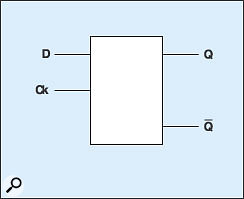Figure 14: The logic symbol for the D flip-flop.The great thing about the JK is that it not only removes the ambiguous state from the Gated RS flip-flop's truth table, it converts it into something useful: a toggle from the existing output value to the next one.

Finally, let's simplify Figures 12 and 13, by adopting logic symbols for the D and JK flip-flops, as shown in Figures 14 and 15, before a quick recap.

## An Audio Delay Line

If you skipped most of this article to get to this point, you've missed some pretty heady stuff. It's your loss, but we can summarise what we've learned as follows:

• The D flip-flop is a device that takes an input, holds it, and passes it to its output.
• The JK flip-flop is a device that allows you to determine the state of its output 'Q', and either store this indefinitely, redetermine it, or toggle it, as desired.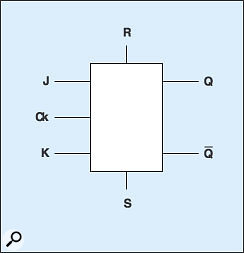Figure 15: The logic symbol for the JK flip-flop.With these two devices, we can now build a one-bit 'shift register' that allows us to determine the value of a single bit at the start of the line, and pass it through numerous elements, as shown in Figure 16.

Of course, in a 16-bit digital audio effects processor (which, of course, is where we came in), a delay line will require 16 such registers

arranged in parallel, fed by the ADC on the input as discussed at the start of this article. At the far end of the delay line, a digital-to-analogue converter (DAC) will convert the data back into an analogue audio signal.

Actually, since the input value of each 'bit' will be determined by the ADC in our effects unit, we don't need to use a JK flip-flop as the first element in the delay line; we can use the simpler D flip-flops throughout (see Figure 17).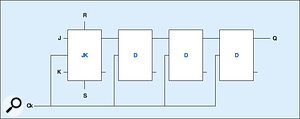Figure 16: A one-bit, four-element, digital delay line.

To demonstrate this, let's consider an example in which the ADC provides a '1' to one of the registers in Figure 17 on the first clock pulse, followed by three '0's. If the rest state of the D flip-flops is '0', the data will pass down the line as shown in Table 11 below.

Naturally, we can expand this concept to include 16-bit information, or 24-bit, or even 64-bit, simply by adding the requisite number of parallel registers, so in principle it is straightforward to pass high-resolution digital audio from the ADC to the DAC.

 ADC D1 D2 D3 D4 Initial state 0 0 0 0 0 Clock 1 1 1 0 0 0 Clock 2 0 0 1 0 0 Clock 3 0 0 0 1 0 Clock 4 0 0 0 0 1 Clock 5 0 0 0 0 0

Table 11: Passing data down a shift register.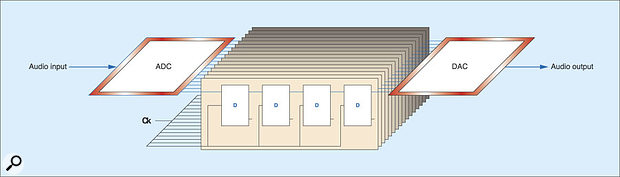Figure 17: A 16-bit digital delay line.

So there we have it... Figure 17 is recognisably equivalent to the BBD shown in Figure 1. As before, the signal enters the delay line through an anti-aliasing filter on the left of the diagram (although, in this case, it's part of the ADC) passes through each stage in turn, and then exits through a reconstruction filter on the right (which, in Figure 17, forms part of the DAC). And, as with the BBD, the length of the delay is determined by just two factors: the number of delay stages, and the speed of the clock that's driving the signal through the devices.

## Epilogue

It is, perhaps, harder to grasp the principles of flip-flops than it is to understand a handful of resistors, capacitors, and analogue switches, but once you have done so, the possibilities become enormous. Depending upon what additional gates and connections are added to the shift register, it is equally at home moving data from right to left as it is left to right, and will accept data in parallel and output it in parallel. Furthermore, if we were to replace all the D flip-flops in the register with JK devices, we could 'program' the value held by each one independently and, at some future point, read back any combination of these values independently. This, of course, is Random Access Memory (RAM). Furthermore, if we were to fix the levels of the inputs to the JKs, the output values would be predetermined, and we have a Read-Only Memory (ROM).

Indeed, the concepts explained this month underlie every aspect of digital technology. From the simplest electronic switches to the 16-, 32- or even 64-bit keyboards, effects units, mixers and computers that we encounter in every walk of our musical lives... they're all based on these ideas.

Let's face it, that's not bad for a bunch of zeros and ones.